# Texas Go Math Grade 3 Lesson 6.4 Answer Key Model with Arrays

Refer to our Texas Go Math Grade 3 Answer Key Pdf to score good marks in the exams. Test yourself by practicing the problems from Texas Go Math Grade 3 Lesson 6.4 Answer Key Model with Arrays.

## Texas Go Math Grade 3 Lesson 6.4 Answer Key Model with Arrays

Essential Question
How con you use arrays to model multiplication and find factors?
Arrays (Rectangles and Area) is another common and important model for multiplication.
Explanation:
An array is a way of arranging the the repeated sets so that you can either count along rows or up and down along columns.
For example,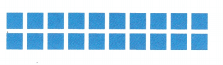2 rows of 10.

Unlock the Problem
Many people grow tomatoes in their gardens. Lee plants 3 rows of tomato plants with 6 plants in each row. How many tomato plants are there?
Explanation:
Lee plants 3 rows of tomato plants with 6 plants in each row.
Total tomato plants 3 x 6 = 18

Activity 1
Materials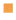square tilesMath Board

• You make an array by placing the same number of tiles in each row, Make an array with 3 rows of 6 tiles to show the tomato plants.
• Now draw the array you made.
• Find the total number of tiles.So, there are _12_ tomato plants.

Math talk
Mathematical Processes
Does the number of tiles change if you turn the array to show 6 rows of 3? Explain.
No, number of tiles wont change.
Explanation:
The number of tiles change if you turn the array to show 6 rows of 3
6 x 3 = 18

Activity 2
Materialssquare tilesMath Board
Use 8 tiles. Make as many different arrays as you can, using all 8 tiles. Draw the arrays. The first one is done for you.

A.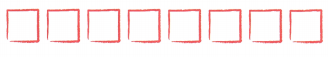1 row of 8
1 × 8 = 8

B.8 rows of __1__
8 x 1 = __ 8
8 × _1_ = 8

C.__ rows of ___
___ × ___ = 8

4 rows of 2
4 x2 = 8

D.__ rows of ___
___ × __ = ____
2 rows of 4
2 x 4 = 8

You can make ___ different arrays using 8 tiles.
Explanation:
A multiplication array is simply an arrangement of rows or columns that matches a multiplication equation.

Share and Show

Question 1.
Complete. Use the array.
___ rows of ___ = ___
___ × ___ = __2 rows of 10 = 20
2 x 10 = 20

Write a multiplication sentence for the array.

Question 2.4 x 6 = 24
Explanation:
4 rows of 6

Question 3.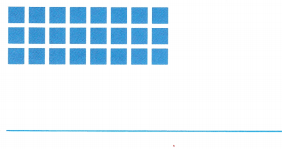3 x 8 = 24
Explanation:
3 rows of 8

Problem Solving

Use the table to solve 4—6.

Question 4.
Representations Mr. Bloom grows vegetables in his garden. Draw an array and write the multiplication sentence to show how many corn plants Mr. Bloom has in his garden.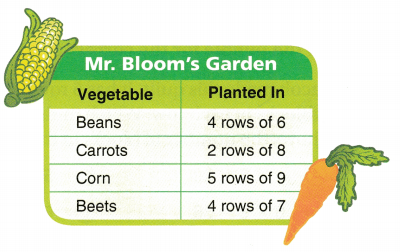5 rows of 9
5 x 9 = 45
Explanation:
Mr. Bloom grows 5 rows of 9 corns.

Question 5.
H.O.T. Write Math Could Mr. Bloom have planted his carrots in equal rows of 4? If so, how many rows could he have planted? Explain.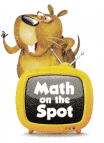4 rows of 4
4 x 4 = 16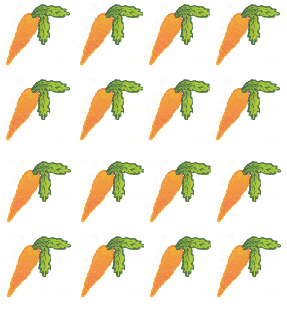Explanation:
2 rows of 8 carrots
Mr. Bloom have planted his carrots in equal rows of 4
So, 4 rows of 4
Question 6.
Multi-Step List the vegetables in order by the number of plants. Put the names in order from greatest to least.Explanation:
Corns 5 x 9 = 45
Beets 4 x 7 = 28
Beans 4 x 6 = 24
Carrots 2 x 8 =16

Question 17.
H.O.T. Mr. Bloom has 12 strawberry plants. Describe all of the different arrays that Mr. Bloom could make using
all of his strawberry plants.1 x 12 =12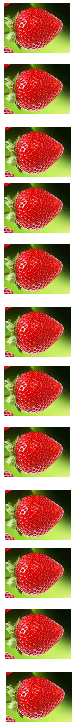12 x 1 = 12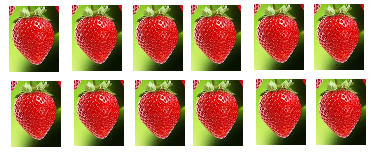2 x 6 = 12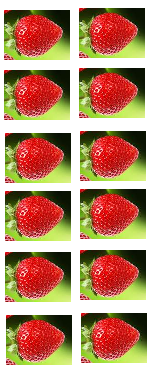6 x 2 = 12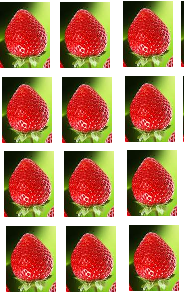4 x 3 = 12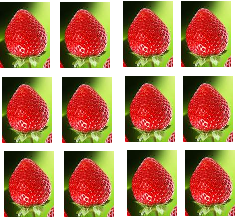3 x 4 = 12
Explanation:
An array is a way of arranging the the repeated sets so that you can either count along rows or up and down along columns.

Fill in the bubble for the correct answer choice.

Question 8.
Jacob made this quilt. It has 5 rows of blocks with 6 blocks in each row. Which multiplication sentence shows the number of blocks in the quilt?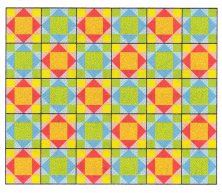(A) 4 × 5 = 20
(B) 6 × 6 = 36
(C) 5 × 6 = 30
(D) 5 × 5 = 25
Option (C)
Explanation:
Jacob quilt has 5 rows of blocks with 6 blocks in each row.
The number of blocks in the quilt
5 x 6 = 30

Question 9.
Apply Karen has 6 animal posters. Which describes one array Karen could make using all of her posters?
(A) 2 rows of 4
(B) 2 rows of 3
(C) 1 row of 5
(D) 3 rows of 3
Option (B)
Explanation:
Karen has 6 animal posters.
Karen can make array using all of her posters
2 rows of 3
2 x 3 = 6 posters

Question 10.
Multi-Step Ashley and Daniel each have a garden with flowers planted in rows. Which multiplication sentence shows the number of flowers in both gardens?(A) 3 × 7 = 21
(B) 4 × 3 = 12
(C) 4 × 4 = 16
(D) 4 × 7 = 28
Option (D)
Explanation:
Ashley ‘s garden has 4 rows of 3 flowers planted =12
Daniel Garden has 4 rows of 4 flowers planted =16
The number of flowers in both gardens
4 x 7 = 28

Texas Test Prep

Question 11.
What multiplication sentence does this array show?(A) 2 × 3 = 6
(B) 4 × 1 = 4
(C) 2 × 5 = 10
(D) 2 × 4 = 8
Explanation:
2 rows of 4

### Texas Go Math Grade 3 Lesson 6.4 Homework and Practice Answer Key

Write a multiplication sentence for the array.

Question 1.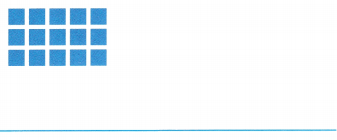3 rows of 5
3 x 5 = 15

Question 2.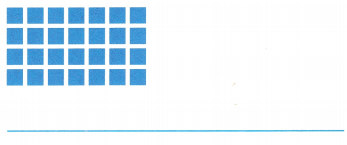4 rows of 7
4 x 7 = 28

Use the table to solve 3-5.

Question 3.
Draw an array and write a multiplication sentence to show how many science books are on Anna’s bookshelves.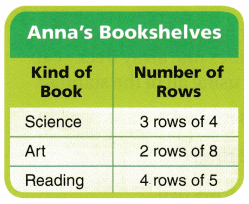3 x 4 = 12
Explanation:
Anna’s bookshelves has 12 science books,
arranged in 3 rows of 4.

Question 4.
Write a number sentence to show how many reading books are on Anna’s bookshelves.
Explanation:
Anna’s bookshelves has 4 rows of 5 reading books.
4 x 5 = 20

Question 5.
Describe three different arrays that Anna can make to show how many art books she has.2). 2 x 8 =16
3). 2 rows of eight.
Explanation:
By drawing array.
By representing in multiplication sentence.
By mentioning the arrangement of rows.

Problem Solving

Question 6.
Sasha makes an array of tiles. She has 3 rows of 9 tiles. Write a multiplication sentence to show Sasha’s array.
3 x 9 = 27
Explanation:
Sasha makes array with 3 rows of 9 tiles.
3 x 9 = 27

Question 7.
Dexter makes an array of tiles. He has 5 rows of 6 tiles. Write a multiplication sentence to show Dexter’s array.
Explanation:
Dexter makes array 5 rows of 6 tiles.
5 x 6 = 30

Texas Test Prep

Lesson Check

Question 8.
Ryan makes this array. Which equation does Ryan’s array show?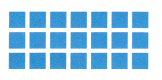(A) 3 × 7 = 21
(B) 3 + 7 = 10
(C) 3 × 6 = 18
(D) 3 × 7 = 20
Option (A)
Explanation:
Each array has 7 tiles,
arranged in 3 rows.

Question 9.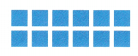(A) 2 + 6 = 8
(B) 6 + 2 = 8
(C) 2 × 6 = 12
(D) 2 × 6 = 26
Option (C)
Explanation:
Total 12 tiles arranged in 2 rows.
Each array has 6 tiles.

Question 10.
Becky has 24 stamps. Which describes one array Becky can make using all of the stamps?
(A) 3 rows of 6
(B) 2 rows of 9
(C) 4 rows of 6
(D) 5 rows of 3
Option(C)
Explanation:
Becky has 24 stamps.
Using all the stamps Becky makes 4 rows

Question 11.
Keesha makes an array of 5 rows of 7 stickers. How many stickers does Keesha have?
(A) 28
(B) 12
(C) 57
(D) 35
Option (D)
Explanation:
Keesha makes an array of 5 rows of 7 stickers.
Total stickers does Keesha have
5 x 7 = 35

Question 12.
Multi-Step DeShawn makes an array using 3 rows of 5 tiles. How many tiles does Deshawn have if he adds 2 more rows to the array?
(A) 15
(B) 9
(C) 20
(D) 25
Option (D)
Explanation:
DeShawn makes an array using 3 rows of 5 tiles.
Deshawn adds 2 more rows to the array.
Total tiles al together = (3 + 2) x 5 = 25

Question 13.
Multi-Step Ming makes an array using 2 rows of 7 tiles. She adds 3 more rows to the array. Which multiplication sentence does Ming’s array show?
(A) 2 × 7 = 14
(B) 3 × 7 = 21
(C) 5 × 7 = 35
(D) 9 × 3 = 27